# Equivalent Fractions

Go back to  'Fractions'

 1 Concept Video on Equivalent Fractions 2 What are Equivalent Fractions? 3 How to find Equivalent Fractions of a Fraction? (with illustration) 4 Think out of the Box! 5 How to determine whether Two Fractions are Equivalent? 6 Equivalent Fractions Chart 7 Important Notes on Equivalent Fractions 8 Activity on Equivalent Fractions 9 Solved Examples on Equivalent Fractions 10 Practice Questions on Equivalent Fractions 11 Equivalent Fractions Worksheets 12 Maths Olympiad Sample Papers 13 Frequently Asked Questions (FAQs)

## Concept Video on Equivalent Fractions

Before we begin explaining about "Equivalent Fractions" in detail, let us first watch this video to see what this topic is all about.

## What are Equivalent Fractions?

Now let us learn about equivalent fractions with the help of its definition and explore a few examples to understand it better.

### Definition of Equivalent Fractions in Math

Two or more fractions are said to be equivalent if all are equal to the same value irrespective of their numerators and denominators.

(or)

Two or more fractions are said to be equivalent if they are all equal to the same fraction when simplified.

### Examples of Equivalent Fractions

Here are some examples of equivalent fractions.

Example 1

$$\dfrac{1}{2}, \dfrac{2}{4}, \dfrac{3}{6}, \text{ and }\dfrac{4}{8}$$ are equivalent fractions.

Let us see how their values are all equal.

We will represent each of the fractions as circles with shaded parts.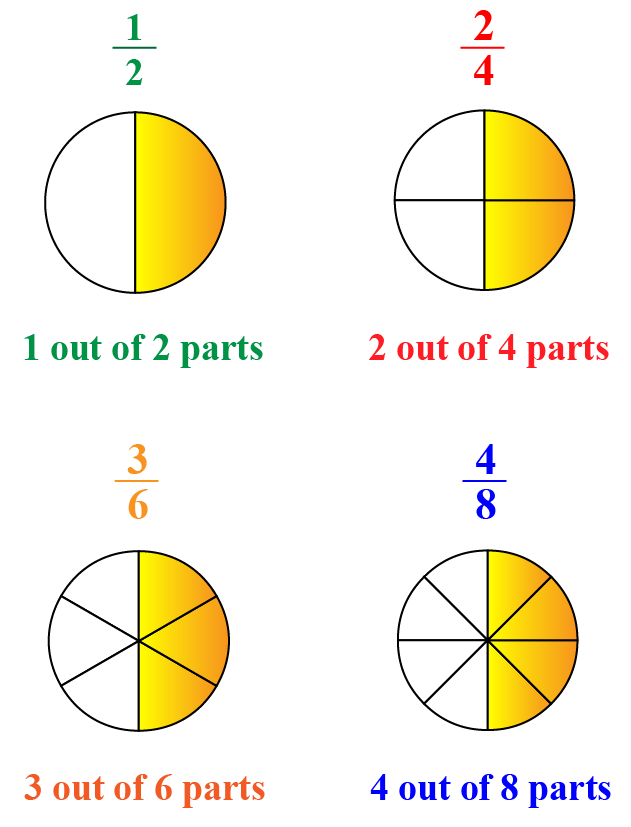Here, we can see that the amount of shaded portion is the same in all the circles.

Hence, $$\dfrac{1}{2}, \dfrac{2}{4}, \dfrac{3}{6}, \text{ and }\dfrac{4}{8}$$ are equivalent fractions.

Example 2

$$\dfrac{1}{3}, \dfrac{2}{6},\text{ and }\dfrac{4}{12}$$ are equivalent fractions.

Let us see how this is true.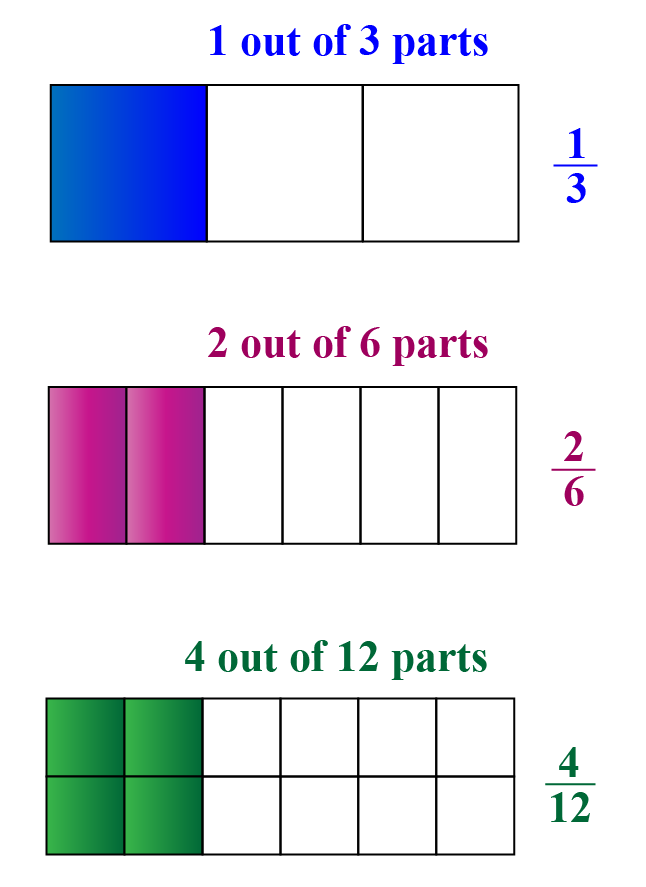Here, we can see that the amount of shaded portion is the same across all the rectangles.

Hence, $$\dfrac{1}{3}, \dfrac{2}{6},\text{ and }\dfrac{4}{12}$$ are equivalent fractions.

## How to find Equivalent Fractions of a Fraction? (with illustration)

The equivalent fractions of a given fraction are found in two ways.

1. By multiplying the numerator and the denominator by the same number
2. By dividing the numerator and the denominator by the same number

### 1. Multiplying the Numerator and Denominator by the Same Number

We will find some equivalent fractions of a fraction $$\dfrac{3}{4}$$

We will find an equivalent fraction for the given fraction by multiplying the numerator $$(3)$$ and the denominator $$(4)$$ by some number, say, $$2$$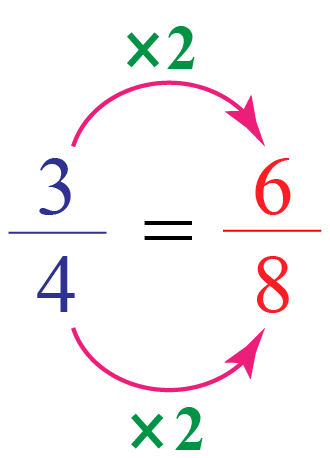Thus, $$\dfrac{6}{8}$$ is an equivalent fraction of $$\dfrac{3}{4}$$

We can find some other equivalent fractions by multiplying the numerator and the denominator of the given fraction by different numbers.

\begin{aligned} \dfrac{3}{4}&= \dfrac{3 \times 3}{4 \times 3} = \dfrac{9}{12}\\[0.3cm] \dfrac{3}{4}&= \dfrac{3 \times 4}{4 \times 4} = \dfrac{12}{16}\\[0.3cm] \dfrac{3}{4}&= \dfrac{3 \times 5}{4 \times 5} = \dfrac{15}{20} \end{aligned}

Thus, the equivalent fractions of  $$\dfrac{3}{4}$$ are:

 $$\mathbf{\dfrac{6}{8}, \dfrac{9}{12}, \dfrac{12}{16}, \dfrac{15}{20},...}$$

We can visualize this method of finding equivalent fractions by the following illustration.

Drag the slider to decide the number with which you want to multiply the numerator and the denominator and see how the two fractions are equivalent visually.

### 2. Dividing the Numerator and Denominator by the Same Number

We can find some equivalent fractions of a fraction $$\dfrac{72}{108}$$

We know that $$2$$ is a common factor of both $$72$$ and $$108$$.

Hence, an equivalent fraction of $$\dfrac{72}{108}$$ can be found by dividing its numerator and denominator by $$2$$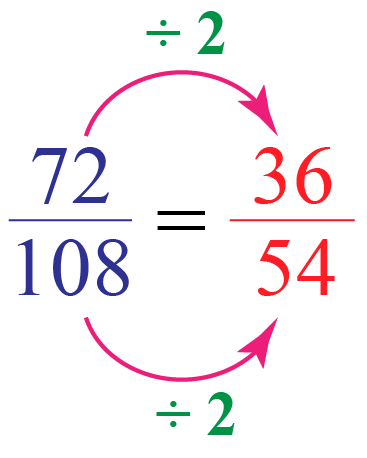Thus, $$\dfrac{36}{54}$$ is an equivalent fraction of $$\dfrac{72}{108}$$

We can also observe that $$2$$ is a common factor of $$36$$ and $$54$$

Hence, another equivalent fraction can be found by dividing its numerator and denominator by $$2$$

\begin{aligned} \dfrac{36}{54}&= \dfrac{36 \div 2}{54 \div 2}= \dfrac{18}{27} \end{aligned}

Again, 3 is a common factor of 18 and 27

\begin{aligned} \dfrac{18}{27}&= \dfrac{18 \div 3}{27 \div 3}= \dfrac{6}{9} \end{aligned}

Again, 3 is a common factor of 6 and 9

\begin{aligned} \dfrac{6}{9}&= \dfrac{6 \div 3}{9 \div 3}= \dfrac{2}{3} \end{aligned}

Thus, some equivalent fractions of $$\dfrac{72}{108}$$ are:

 $$\mathbf{\dfrac{36}{54}, \dfrac{18}{27}, \dfrac{6}{9}, \dfrac{2}{3},...}$$

Here, $$\dfrac{2}{3}$$ is the simplified form of $$\dfrac{72}{108}$$ as there is no common factor (other than 1) of $$2$$ and $$3$$Think Tank
1. Can we multiply the numerator and denominator of a fraction by a decimal to get its equivalent fraction?
2. Can we divide the numerator and denominator of a fraction by a decimal to get its equivalent fraction?

## How to determine whether Two Fractions are Equivalent?

There are various methods to identify whether the given fractions are equivalent.

Some of them are:

1. Making the denominators (or numerators) the same
2. By finding the decimal equivalent of both fractions
3. Cross multiplication method
4. Visual method

Let us identify whether $$\dfrac{2}{6} \text{ and }\dfrac{3}{9}$$ are equivalent fractions in each of these methods.

### 1. Making the Denominators the Same

The denominators of the fractions $$\dfrac{2}{6}, \text{ and }\dfrac{3}{9}$$ are $$6$$ and $$9$$

The LCM of the denominators $$6$$ and $$9$$ is $$18$$

Let us make the denominators of both fractions $$18$$ by multiplying them with suitable numbers.

\begin{aligned} \dfrac{2}{6} &= \dfrac{2 \times 3}{6 \times 3}= \dfrac{6}{18}\\[0.3cm] \dfrac{3}{9} &= \dfrac{3 \times 2}{9 \times 2}= \dfrac{6}{18} \end{aligned}

We can observe that both fractions are equivalent to the same fraction $$\dfrac{6}{18}$$

Thus, the given fractions are equivalent.

Note: If the fractions are NOT equivalent, we can see which is the greater (or lesser) fractions by looking at the numerator of both resultant fractions in this method.

Hence, this method is used to compare the fractions as well.

### 2. Finding the Decimal Equivalent of Both Fractions

Let us find the decimal equivalent of both fractions $$\dfrac{2}{6} \text{ and }\dfrac{3}{9}$$ and see whether they both give the same value.

\begin{aligned}\dfrac{2}{6} &= 0.3333333...\\[0.3cm] \dfrac{3}{9} &= 0.3333333... \end{aligned}

The decimal values of both fractions are the same and hence, they are equivalent.

### 3. Cross Multiplication Method

To identify whether $$\dfrac{2}{6} \text{ and }\dfrac{3}{9}$$ are equivalent, we cross multiply them.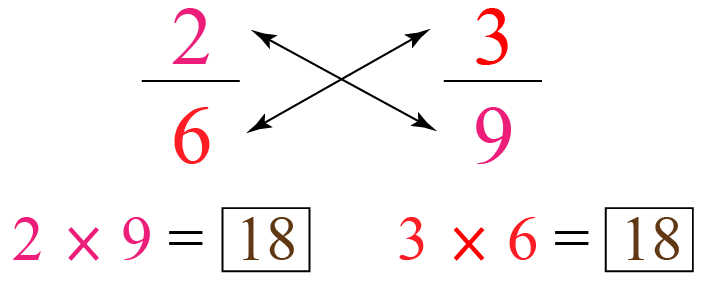If both products are the same, the fractions are equivalent.

Since both products here are the same $$(18)$$, the given fractions are equivalent.

### 3. Visual method

Let us represent each of the fractions $$\dfrac{2}{6} \text{ and }\dfrac{3}{9}$$ pictorially on identical shapes and check whether the shaded portions of both are equal.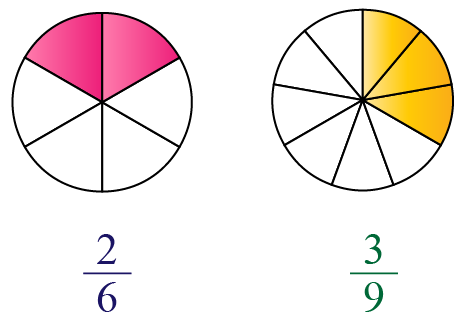We can see that the shaded portions of both circles are equal.

Hence, the given fractions are equivalent.

## Equivalent Fractions Chart

Let us use the following chart to find the equivalent fractions of $$\dfrac{1}{4}$$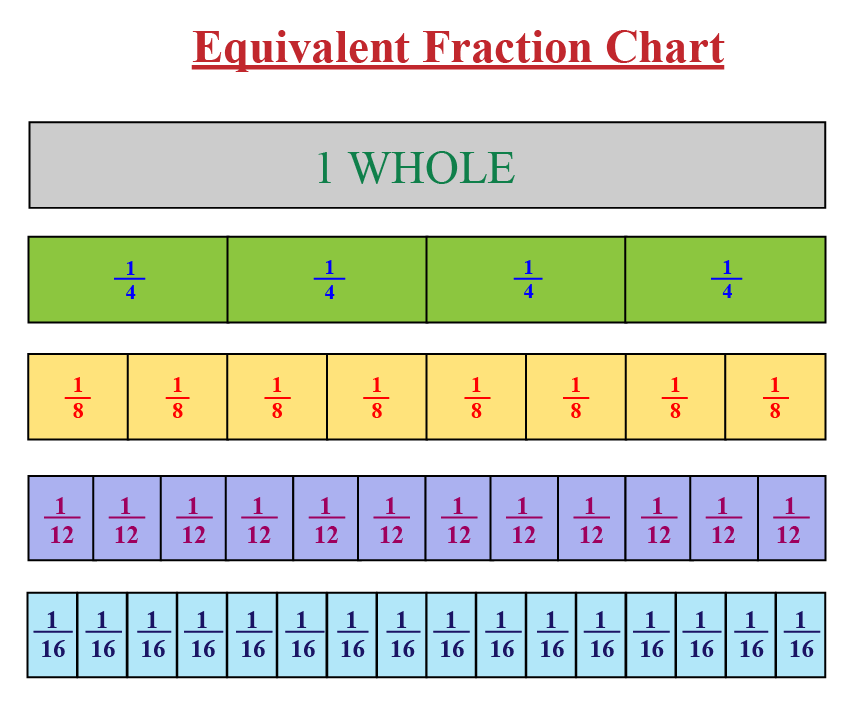From this chart, we observe that the equivalent fractions of $$\dfrac{1}{4}$$ are:

 $$\mathbf{\dfrac{2}{8}, \dfrac{3}{12}, \dfrac{4}{16},...}$$

## Solved Examples

 Example 1

Ryan gave $$\dfrac{2}{5}$$ of an apple to Mary and $$\dfrac{7}{10}$$ of another apple that was same in size to John.

Did Ryan give the same amount of apple to each of them?

Solution:

Amount of apple that Ryan gave to Mary = $$\dfrac{2}{5}$$

Amount of apple that Ryan gave to John = $$\dfrac{7}{10}$$

To check if Ryan gave the same amount of apple to both of them, we have to see whether these two fractions are equivalent.

Since we have only two given fractions, we will go for the cross multiplication method.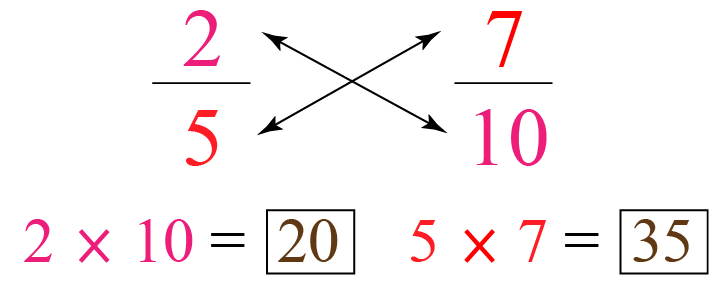Since both products $$(20 \text{ and } 35)$$ are different, the given fractions are NOT equivalent.

Note: Here, $$20 < 35$$. Thus, we can say that Ryan gave a bigger share of the apple to John.

Thus,

 Ryan did NOT give the same amount of apple to Mary and John
 Example 2

If the numerator of the fraction equivalent to $$\dfrac{9}{27}$$ is $$27$$, what is its denominator?

Solution:

We know that,

$\dfrac{9}{27}=\dfrac{27}{\square}$

Here, the numerators are $$9$$ and $$27$$

Now, we have to find a number which when multiplied by $$9$$ gives $$27$$

We know that $$9 \times 3 = 27$$

Hence, we multiply the numerator and the denominator of the given fraction by $$3$$

$\dfrac{9}{27} = \dfrac{9 \times 3}{27 \times 3}= \dfrac{27}{81}$

Thus, the denominator of this equivalent fraction is:

 $$\mathbf{81}$$

## Practice Questions

Here are few activities for you to practice. Select/Type your answer and click the "Check Answer" button to see the result.

## Equivalent Fractions Worksheets

We can find equivalent fractions worksheets at the bottom of this page.

Make use of the worksheets to understand the equivalent fractions better.

IMO (International Maths Olympiad) is a competitive exam in Mathematics conducted annually for school students. It encourages children to develop their math solving skills from a competition perspective.

## 1. What are equivalent fractions?

Two or more fractions are said to be equivalent if all are equal to the same value irrespective of their numerators and denominators.

## 2. What are the examples of equivalent fractions?

Example 1: $$\dfrac{2}{3}$$ and $$\dfrac{6}{9}$$ are equivalent fractions.

Example 2: $$\dfrac{4}{7}$$ and $$\dfrac{28}{49}$$ are equivalent fractions.

You can find more examples under "What are equivalent fractions?" section of this page.

## 3. How to determine whether fractions are equivalent?

There are various methods to identify whether the given fractions are equivalent.

Some of them are:

1. Making the denominators (or numerators) the same
2. By finding the decimal equivalent of both fractions
3. Cross multiplication method
4. Visual method

You can learn each method in detail by going through the section, "How to Determine Whether Two Fractions are Equivalent?", of this page.

More Important Topics
Numbers
Algebra
Geometry
Measurement
Money
Data
Trigonometry
Calculus